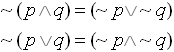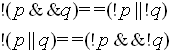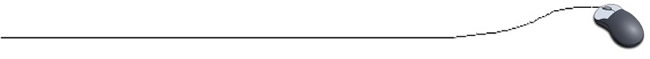Logical Operators

Programmers need to know the rules for mathematical logic.
We will actually be putting logic and even truth tables to use as we are programming. Check them out below:

 Mathematical Logic AND AND is true only when BOTH statements are true. OR OR is true when either (or both) statements are true. NOT NOT is true when the statement is false. Think opposite.

 Computer Logic (logical operators) Logical AND The symbol is &&. Example: (x > 4) && (x < 9) Logical OR The symbol is | |. Found above the backslash on the keyboard. Example: (a < 3) | | (a > 10) Logical NOT The symbol is !. Example: !(4 + 3 = 5) is true.
 A single comparison in an if statement often is not enough to determine whether data matches your criteria.  For example, you may need to check to see if a user enters a number within a certain range.  To accomplish this task, you will need the logical operators in your if statement.  These logical operators which result in a true/false condition are also referred to as Boolean Operators.

Check out the TRUTH VALUE of these statements using logical operators:

 && (AND) (3 + 2 = 5) && (6 + 2 = 8)   TRUE (4 + 3 = 9) && (3 + 3 = 6)   FALSE | | (OR)   found above the backslash on the keyboard (3 + 6 = 2) || (4 + 1 = 5)      TRUE (1 + 1 = 3) || (3 + 3 = 9)      FALSE ! (NOT) !(4 + 3 = 5)      TRUEBEWARE: Math:             2 < x < 10    OK Computer:    if (2 < x < 10)   NO!!!                        Will only do the first condition.               if ((2 < x) && (x < 10))  YES

For all the math nerds (like us), know that programmers also use and understand DeMorgan's Laws:

 Remember DeMorgan's Laws: Mathematical Version: Programming Version:Equivalent statements when programming: if (!(num >= 0 && num <= 65)) if (num < 0 || num > 65)